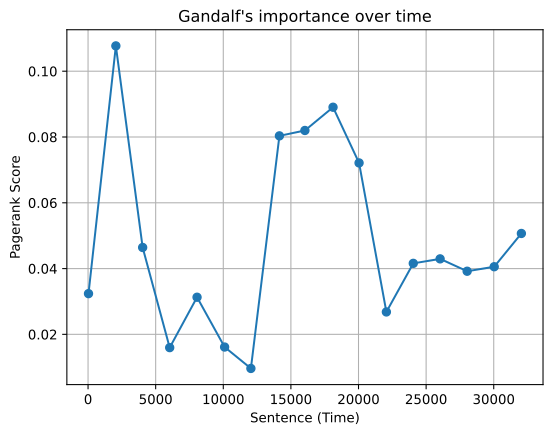# Running algorithms on graph views

As with all the queries we saw in the previous chapter, both `graphwide` and `vertex centric` algorithms can be run on `graph views`. This allows us to see how results change over time, run algorithms on subsets of the layers or remove specific vertices from the graph to see the impact this has.

To demonstrate this, below is an example of how you could track Gandaf's importance over the course of the story using rolling windows and the `PageRank` algorithm.

Within each windowed graph we use the AlgorithmResult api to extract Gandalf's score and record it alongside the earliest timestamp in the window, which can then be plotted via matplotlib.

``````# mkdocs: render
import matplotlib.pyplot as plt
import pandas as pd
from raphtory import algorithms as rp
from raphtory import Graph

lotr_graph = Graph.load_from_pandas(edges_df=df, src="src", dst="dst", time="time")

importance = []
time = []

for windowed_graph in lotr_graph.rolling(window=2000):
result = rp.pagerank(windowed_graph)
importance.append(result.get("Gandalf"))
time.append(windowed_graph.earliest_time())

plt.plot(time, importance, marker="o")
plt.xlabel("Sentence (Time)")
plt.ylabel("Pagerank Score")
plt.title("Gandalf's importance over time")
plt.grid(True)
``````